##### GMAT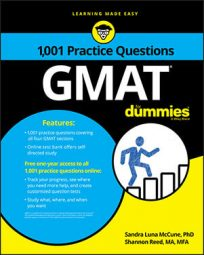Some Problem Solving questions in the Quantitative section of the GMAT will involve geometry. You should know how to work with angles, lines, two-dimensional shapes, three-dimensional solids, perimeter, area, surface area, volume, the Pythagorean theorem, and coordinate geometry.

## Practice questions

The figure shows a triangle inscribed in a semicircle.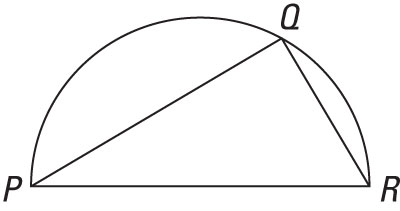1. If PQ = 16 and QR = 12, what is the length of arc PQR?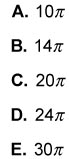The figure shown is a rhombus in which the measure of angle A is 120 degrees.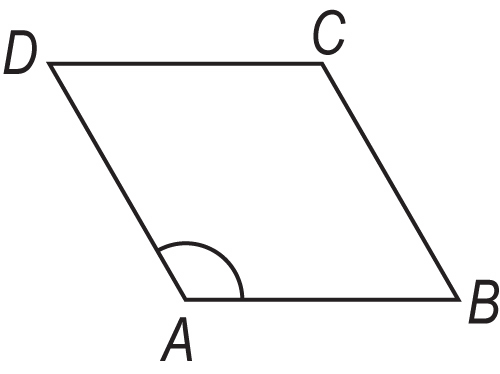2. What is the ratio of the length of line AC to the length of line DB?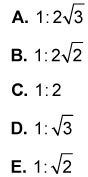1. The correct answer is A.

An angle inscribed in a semicircle is a right angle. Thus, triangle PQR is a right triangle with legs of lengths 16 and 12. The length, PR, of the hypotenuse is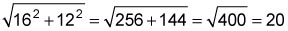Thus, the diameter of the semicircle is 20. The length of the arc PQR is half the circumference of the circle that contains the semicircle. This length is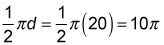2. The correct answer is D.

Consecutive interior angles of a rhombus are supplementary, so the measure of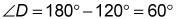The diagonals of a rhombus are perpendicular bisectors of each other and bisect the angles of the rhombus. Construct the diagonals of the rhombus. Label the intersection E.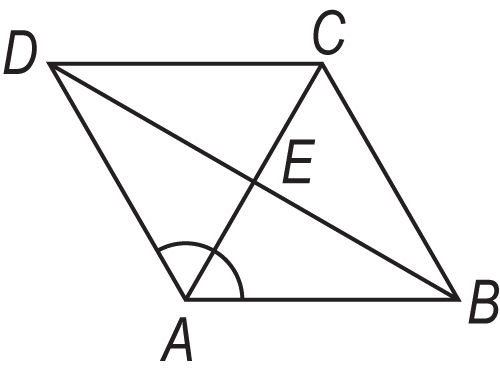Thus, triangle AED is a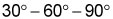right triangle, with hypotenuse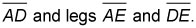Given that the diagonals bisect each other, the length of line AC is twice the length of line AE, and the length of line DB is twice the length of line DE. Hence, the ratio of the length of line AC to the length of line DB is the same as the ratio of the length of line AE to the length of line DE. The lengths of the sides of a 30 – 60 – 90 right triangle are in the ratio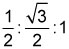Therefore, the ratio of the length of line AC to line DB equals the ratio of the length of line AE to line DE equals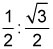which is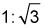in simplified form.